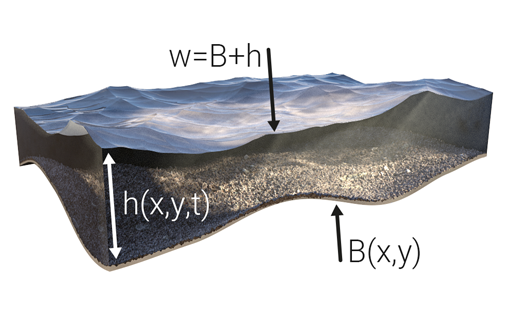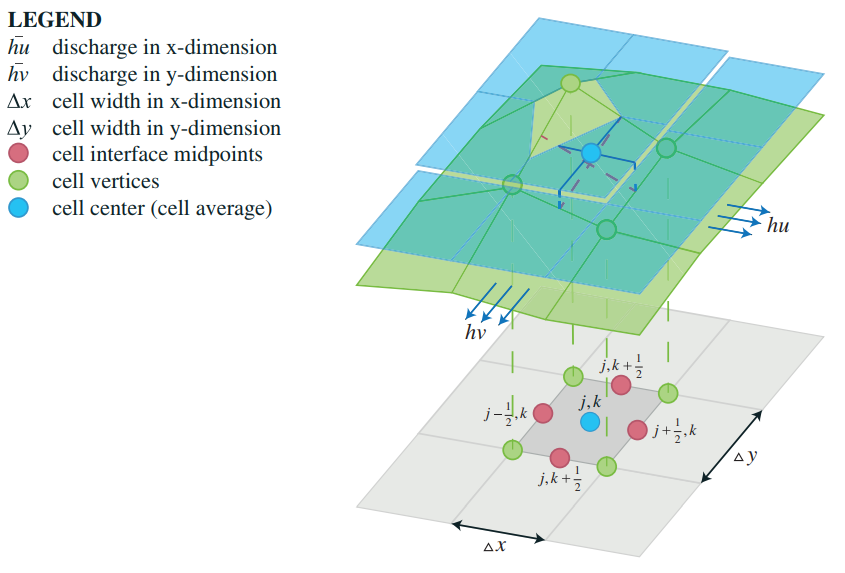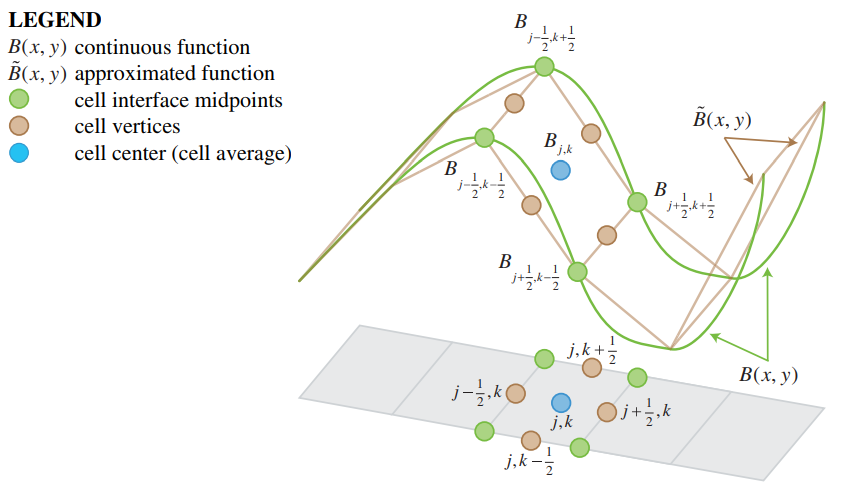# Surface model (Water Overlay)Fig. 1. For each grid cell:
B = bottom elevation
h = water depth
w = water surface elevationFig. 2. A 2-D grid-based representation of average water depths, discharges and bathymetry in a case where the middle cell is fully flooded in the y-dimension, but only partially flooded in the x-dimension. Blue solid and red dashed lines denote the waterlines and bathymetry slopes in both dimensions, respectively. For a bilinear reconstruction, the cell averages coincide with the values at the cell centers, while bathymetry is approximated by its values at the cell vertices.Fig. 3. Continuous bathymetry function (green line) and its piecewise linear approximation (brown dots). The approximated function values equal to the continuous ones at the cell vertices (green dots). The cell average value (blue dot) equals both the average value of the vertex values (green dots) and the average value of the values at the cell interface midpoints (brown dots).

The Water Module's primary function is simulating two-dimensional flow of water across the surface. In order to do this, the project area is first discretized into x by y cells depending on the configured grid cell size. Secondly, water is initialized in the model. Finally, a set of rules is required that describes the behavior of the flow.

### Water level initialization

In theory, each grid cell can have a unique bottom elevation and accompanying water depth, yielding a certain water surface elevation (or water level). However, in practice, water levels are often initialized for large groups of cells rather than individual cells, assuming that each (water level) area in a project has been assigned a water level. During the initialization phase a distinction is made between two types:

For all water terrains in a water area, the volume of water per grid cell is such that the resulting water level at those locations conforms the value of the WATER_LEVEL attribute as provided by the corresponding water area.

In contrast to water areas, which generate water on water terrains, inundation areas generate water over all grid cells, regardless of its terrain type. Similarly, the volume of water per grid cell is determined by the INUNDATION_LEVEL attribute as provided by the corresponding inundation area.

If a grid cell is neither part of any water area nor inundation area, or the assigned water level is lower than its bottom elevation, the water depth is assumed to be zero and the water level becomes equal to the bottom elevation. In turn, this bottom (or surface) elevation is equal to the elevation model, though it may be altered by the presence of a construction or the BREACH_HEIGHT attribute of a breach.